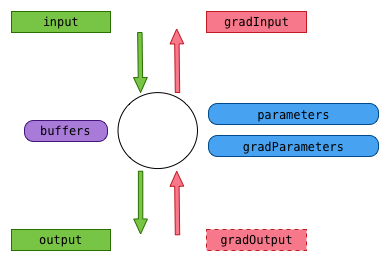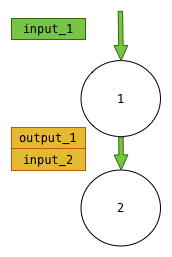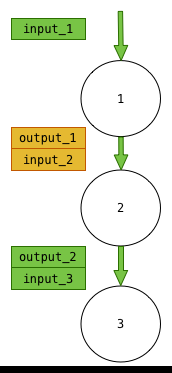# Further Memory Optimization

This post is sharing some notes on how memory optimization is done in OpenNMT and list tracks to have better memory optimization especially for larger training. Control of memory is especially important when training on GPU since memory is limited - and this limitation force smaller sequence length (which is is impossible for tasks like summarization), or smaller batches which reduces convergence speed.

Feel free to contribute if you have ideas.

### Memory Usage

A sequence-to-sequence model includes several models that are represented as a computation graph:

• the encoder (recurrent network)
• the decoder (recurrent network)
• the bridge (between encoder and decoder)
• the generator (not recurrent)

Each computation graph is a sequence of operations (nodes) applying on inputs (`input`) and generating outputs (`output`) possibly using parameters (generally `weight` and `bias`) when applied in forward mode, and in backward mode generating gradients on parameters (`gradParams`) and inputs (`gradInput`) from output gradient (`gradOutput`). Also some nodes will use internal `buffers` (for instance noise in a dropout cell) to store temporary information that is being used for the forward and the backward passes.Inputs, outputs, parameters, their gradients and the buffers can be tensor or table of tensors.

Parameters are permanently stored with the nodes, inputs and outputs are also stored in the computation node because we need to keep track of previous intermediate calculations in forward mode and we need also persistency between forward and backward passes. `gradOutput` does not have to be stored because only used once during backward propagation.

All these tensors are using the GPU memory and to optimize memory usage, we do need to share the storage blocks they are using which can be done in different ways.

#### Sharing parameters

For training a recurrent network, the nodes have to replicated in multiple timesteps, so that backpropagation can apply at each timesteps, but `parameters` and `gradParameters` are shared between all the different replicas.

For inference, the computation graph can be re-used for each timestep, since there is no need to remember the stages of \$t-1\$ when calculating \$t\$

#### Connecting outputs and inputs

Another obvious sharing is to use only one storage for input/output when 2 nodes are connected. In the following diagram, we can see that we can safely merge the output of the first node with the input of the second nodes: they are exactly the same.#### Vertical sharing

The third way is to recycle some of the storages when there is no dependency between them. For instance in the following diagram we represent that the `output_2`/`input_3` tensors are using the same storage than `input_1`:It is farily intuitive if we consider an calculation path - for instance, to calculate \$(1+3)*5+2\$, it is clear that we can use the storage used to number 3 to store the result of calculation of the complete expression.

However, this can be done only if:

1. in the forward pass, `input_1` is not re-used for another operation down the computation graph
2. in the backward pass: if the calculation of `gradInput_1` does not require to know the value of `input_1` or `output_1`. Some modules, like `Linear` calculate `gradInput` using `gradOutput` but also `input`.
3. the size of the 2 storages are compatible

#### Horizontal sharing

The last way to sharing is between replicas as long as the condition (2) above is respected. This is a very efficient sharing since it repeats for each additional replica allowing to extend the number of replicas. The following diagram shows case, where for some reason `input_1` at each timestep can not be shared, but `input_2` and `input_3` can be shared.

#### Local tricks

Note that some “tricks” can sometime be applied locally when we know for instance that the output buffer can reuse input buffers - for example, a module adding a constant to an input buffer does not need to keep value of the input for the backpropagation.

### Implementation status

The lua implementation of OpenNMT (up to version 0.7) implements horizontal memory sharing.

### External references

• the excellent optnet torch library written by Francisco Massa is implement vertical sharing strategies on torch `nn` graphs.
2 Likes